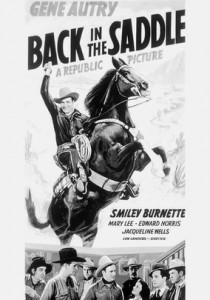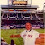## Monday, January 21, 2013

### NFL Picks Contest - Results and Updated StandingsMOJO is back in the saddle again.
Here are the results and updated standings in the NFL Picks Contest.

It looks like a two horse race going into the Super Bowl. Vegas Vic will have to try to not only guess which team will win, but also which team MOJO will pick. If both pick the same team, MOJO will be getting fitted for his new shirt!

The Pro Bowl is this weekend, but will not be counted in our contest since it's basically a glorified practice game.

lightning- San Francisco (W 28-24) = 14 pts.
TwoMinuteWarning- New England (L 13-28) = <15> pts.
Grange95-  San Francisco (W 28-24) = 14 pts.
Music City Momma- New England (L 13-28) = <15> pts.
Memphis MOJO-  San Francisco (W 28-24) = 14 pts.
Vegas Vic-  New England (L 13-28) = <15> pts.
Captain Crunch- Atlanta (L 24-28) = <4> pts.

Here are the current standings;

Memphis MOJO - 40+19+22+13+16+18+24+68+22+17+45+24-(3)+14 = 339 pts.
Vegas Vic - 16+19+22+13+16+40+15+23+43+58+24+24+23-(15) = 321
TwoMinuteWarning - 13+19+22+16+31+18+19+23+31+58+24+24+23-(15) =306
lightning - 13+19+24+13+16+18+24+24+22+17+38+24+23+14 =289
Music City Momma - 17+19+24+5+17+34+24+24+43+17+24+24+23-(15) = 280
Grange95 - 20+19+22+13+14+40+24+23+43+17+45-(15)-(3)+14 = 276
Captain Crunch - 13+11+18+16+31+18+24-(2)+31+32+24+20+23-(4) =255

Good luck!

1.2.